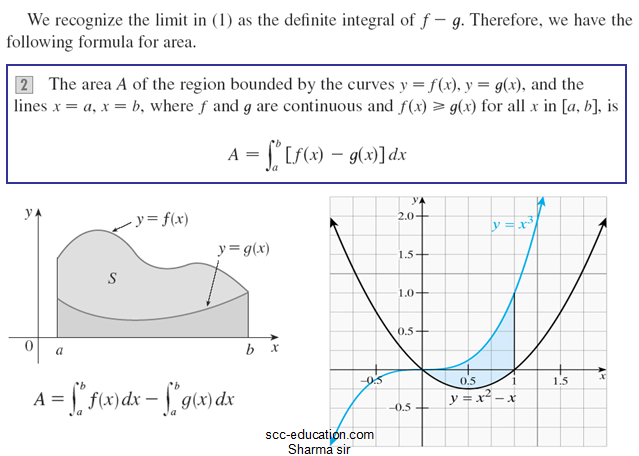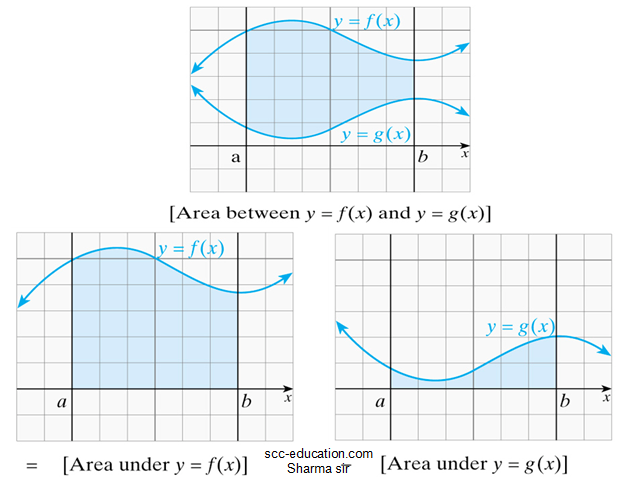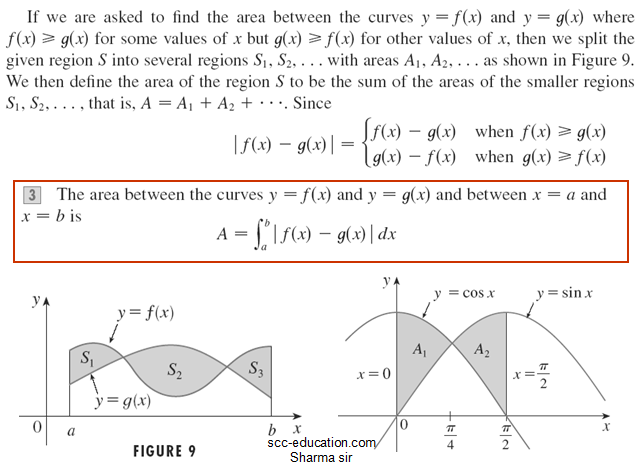# SCC Education

## Applications of Integration

Applications of Integration
we explore some of the applications of the definite integral by using it for
Computing the area between curves,
Computing the volumes of solids,
Computing the work done by a varying force,
Computing average value of a function,
The common theme is the following general method, which is similar to the one we used to find areas under curves:
We break up a Q quantity into a large number of small parts. We next approximate each small part by a quantity of the form              and thus approximate Q by a Riemann sum. Then we take the limit and express Q as an integral Finally we evaluate the integral using the Fundamental Theorem of Calculus or the Midpoint Rule.some-important-formulas-of-integration
solving-models-related-to-ordinary differential equation
differentiation-of-exponential-functions
area-between-curve
vector
application-of-derivatives
homogenous-differential-equation
inverse-trigonometry-questions
integration
integration-questions
Rolles-theorem-and-mean-value-theorem
Techniques-of-integration-substitution
critical-points-and-point-of-inflection

intermediate-value-theorem